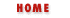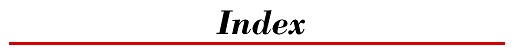Symbols | A | B | C | D | E | F | G | H | I | J | K | L | M | N | O | P | Q | R | S | T | U | V | W | X | Y

# M

Macintosh platforms : Window.open() method
data tainting on : Enabling Data Tainting in Navigator 3.0
mailing list on JavaScript : Discussion of JavaScript
mailto: protocol specifier
Security Holes and Security Hobbles
Security Hobbles in Navigator 3.0
manipulating images (see images)
<MAP> tags : The Link Object
Math object
Arithmetic and Mathematical Functions
Math
Math.abs()
Math.acos()
Math.asin()
Math.atan()
Math.atan2()
Math.ceil()
Math.cos()
Math.E
Math.exp()
Math.floor()
Math.LN10
Math.LN2
Math.log()
Math.LOG10E
Math.LOG2E
Math.max()
Math.min()
Math.PI
Math.pow()
Math.random()
Math.round()
Math.sin()
Math.sqrt()
Math.SQRT1_2
Math.SQRT2
Math.tan()
mathematical functions : Arithmetic and Mathematical Functions
arithmetic operators
Arithmetic and Mathematical Functions
Arithmetic Operators
computation : Still More Features
max() : Math.max()
MAX_VALUE constant : Number.MAX_VALUE
MAYSCRIPT attribute : The MAYSCRIPT attribute
memory
management in Navigator 2.0 : Per-Page Memory Management in Navig...
reclaiming (see garbage collection)
setTimeout() bug : setTimeout() memory leak
+ operator and : Workarounds for Navigator 2.0
method property
Form method property
Form.method
methods
Functions
Methods
instance methods : Classes in JavaScript
JavaMethod object
The JavaMethod Object
Java Field Values versus Method Ret...
JavaMethod
for manipulating arrays : Array Methods
special, for every object : Special Object Methods
static (class) : Classes in JavaScript
tainting : Data Tainting in JavaScript
Microsoft's ActiveX : Calling JavaScript from Applets
milliseconds (see date and time; seconds)
MIME types : Non-HTML Documents
MimeType object
Miscellaneous Changes
The MimeType Object
MimeType
MimeType.description
MimeType.enabledPlugin
MimeType.suffixes
MimeType.type
mimeTypes[] property
Still More Features
Navigator.mimeTypes[]
for Navigator object : The MimeType Object
min() : Math.min()
minus (-) operator : Subtraction (-)
minutes
Date.getMinutes()
Date.setMinutes()
MIN_VALUE constant : Number.MIN_VALUE
modulo (%) operator : Modulo (%)
month : Date.setMonth()
months : Date.getMonth()
more than operator (see greater than operator)
MorphObject() : Live3D
mouse events (see event handlers; onClick(); onMouseOver())
movies (see LiveVideo plug-in)
multidimensional arrays : Multidimensional Arrays
multiple
browser windows : Multiple Windows and Explicit Windo...
running scripts : JavaScript and Threads
scripts in document : The <SCRIPT> Tag
multiplication (*) operator : Multiplication (*)